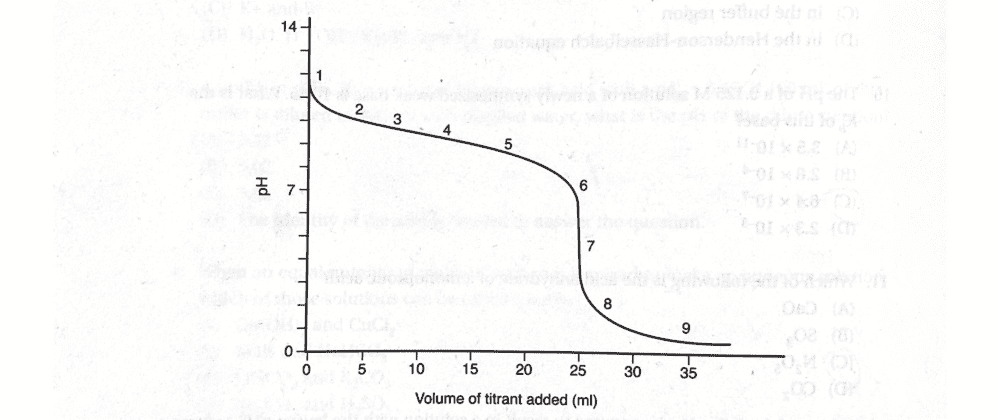# PKb of weak base titrated with strong acid

i_love_science
Homework Statement:
(see below)
Relevant Equations:
titration curve
pH + pOH = 14

at halfway point:
pH=pKa
pOH=pKbWhat is true about the pK of the analyte?
a) pKa = 9.2
b) pKb = 9.2
c) pKb = 4.8
d) pKa = 11.7

The halfway point is at point 4, at approximately pH=9.2. Therefore, the pOH = pKb = 4.8, and I think the answer is c).
The solution says b) is the correct answer. Could anyone explain why, or whether or not my answer is correct? Thanks.